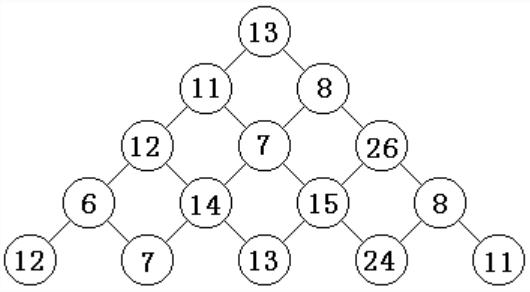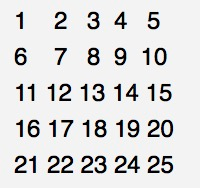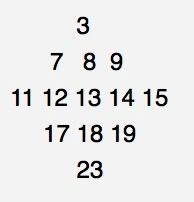01/09 12:09

# 数字三角形+字母塔+字母表+Matrix+ Jumping Frog（跳蛙）+求两圆相交的面积+看电影+谷歌的招聘+汉诺塔问题+表达式求值

7-1 数字三角形 (10分)5
13
11 8
12 7 26
6 14 15 8
12 7 13 24 11

86

#include<iostream>
using namespace std;
int a;//存放数塔
int main()
{

int n;
cin>>n;
for(int i=1;i<=n;i++)
{

for(int j=1;j<=i;j++)cin>>a[i][j];
}
for(int i=n-1;i>=1;i--)
{

for(int j=1;j<=i;j++)
{

a[i][j]+=max(a[i+1][j],a[i+1][j+1]);//自底向上找最大值更新数塔
}
}
cout<<a;
return 0;
}


7-2 字母塔 (10分)

高度(不超过 26 的正整数)


显示指定高度的字母塔


5

A


ABA
ABCBA
ABCDCBA
ABCDEDCBA

//其实很简单，多试几次就好了
#include<iostream>
using namespace std;
int main()
{

int n;
cin>>n;
for(int i=1;i<=n;i++)
{

char x='A';
for(int j=n-i;j>0;j--)cout<<" ";
for(int j=1;j<=i;j++)
{

cout<<x;
x=x+1;
}
x=x-1;
for(int j=1;j<i;j++)
{

x=x-1;
cout<<x;
}
cout<<endl;
}
}


7-3 字母表 (10分)

2
xyzabcdefghijklmnopqrstuvw
aiemckgobjfndlhp

3
20

//当作最长上升子序列
#include <bits/stdc++.h>
#define ll long long
using namespace std;
string q;
ll dp;
int main()
{

int t;
cin>>t;
while(t--)
{

cin>>q;
for (int i=0;i<105;i++)
{

dp[i] = 1;
}
for (int i=0; i <q.size(); i++)
{

for (int j=0;j<i;j++)
{

if (q[i]>q[j])
{

dp[i]=max(dp[i], dp[j]+1);
}
}
}
sort(dp,dp+105);
cout<<26-dp<<endl;
}
return 0;
}


7-4 Matrix (10分)

Give you an N * N matrix, fill in the number of 1 to N * N in order, for example N = 5, the matrix is as followsNow let you connect the midpoints of the adjacent two sides, and then only keep the numbers they enclose in the closed graphics area, then the matrix becomesNow ask you for the sum of all the elements of the changed matrix.

Input the first line of an integer T (1 <= T <= 100) Next, there is a group test data, and each set of test data is input with an integer N (3 <= N <= 10000) Guaranteed input n is odd

For each set of test data, output the corresponding answer

2
3
5

25
169

//找规律水题
#include<iostream>
using namespace std;
int main()
{

long long  n;
cin>>n;
while(n--)
{

long long  m;
cin>>m;
cout<<(1+m*m)/2*(1+m*m)/2<<endl;
}
}


7-5 Jumping Frog（跳蛙） (10分)

A frog is located at the coordinate (x1,y1). He wants to go to the coordinate (x2,y2). He will perform one or more jumps to reach his destination. The rule of the jumping is as follows:

Suppose the frog is located at the coordinate (x,y); then he can jump to the following foursquares:

(x+y,y)
(x-y,y)
(x,y+x)
(x,y-x)


The Problem: (存在的问题:)

Given the coordinates (x1,y1) and (x2,y2), you need to determine if it is possible for the frog to travel from (x1,y1) to (x2,y2) through a series of jumps as described.

The Input:

The first input line contains an integer, n (1 ≤ n ≤ 100), indicating the number of test cases.

Each test case consists of four integers (between -1,000,000,000 to +1,000,000,000 inclusive) separated by a single space denoting x1, y1, x2 and y2, respectively.

The Output:

For each test case, output 1 if the frog can travel from (x1,y1) to (x2,y2) through a series of jumps as described or 0 otherwise.

Sample Input:

3
-6 8 17 25
13 17 -16 11
0 0 5 6

Sample Output:

0
1
0

//由题意知他每次能移动的位移是其坐标的最大公因数的k倍，所以只要最大公因数相同即可
#include <bits/stdc++.h>
typedef long long ll;
using namespace std;
int gcd(ll a,ll b)
{

if(b==0)return a;
else return gcd(b,a%b);
}//gcd求最大公因数
int main()
{

ll n,x1,x2,y1,y2;
cin>>n;
while(n--)
{

cin>>x1>>y1>>x2>>y2;
if(gcd(abs(x1),abs(y1))==gcd(abs(x2),abs(y2)))cout<<1<<endl;
else cout<<0<<endl;
}
return 0;
}


7-6 求两圆相交的面积 (10分)

0 0 1 2 2 1

2 0 2 5 2 3

0.00

3.22

#include<iostream>
#include<math.h>
using namespace std;
int main()
{

int x1,y1,r1,x2,y2,r2;
cin>>x1>>y1>>r1>>x2>>y2>>r2;
double d=sqrt(pow((x1-x2),2)+pow((y1-y2),2));
if(d>=r1+r2)printf("%.2lf",0);//外切和相离
else if(d<=r1-r2)printf("%.2lf",acos(-1.0)*r2*r2);//两圆内切，r2小
else if(d<=r2-r1)printf("%.2lf",acos(-1.0)*r1*r1);//两圆内切，r1小
else//相交
{

double angle1=acos((r1*r1+d*d-r2*r2)/(2*r1*d));//左扇区对应角度
double angle2=acos((r2*r2+d*d-r1*r1)/(2*r2*d));//右扇区对应角度
double s1=angle1*r1*r1;//左扇区对应面积
double s2=angle2*r2*r2;//右扇区对应面积
double s3=r1*d*sin(angle1);//四边形面积
printf("%.2lf",s1+s2-s3);
}
}


7-7 看电影 (10分)

12
1 3
3 4
0 7
3 8
15 19
15 20
10 15
8 18
6 12
5 10
4 14
2 9
0

5

#include<iostream>
#include<algorithm>
using namespace std;
struct film
{

int a;
int b;
};
bool cmp(film x, film y)
{

return x.b < y. b;
}
int main()
{

int n;
while(cin>>n)
{

if(n==0)break;
int sum=0,i;
film f;
for(i=0;i<n;i++)cin>>f[i].a>>f[i].b;
sort(f,f+n,cmp);//对结束时间进行排序
int m=0;
for(i=0;i<n;i++)
{

if(f[i].a>=m)//从启示时间遍历
{

sum++;
m=f[i].b;
}
}
cout<<sum<<endl;
}
return 0;
}


7-8 谷歌的招聘 (10分)

2004 年 7 月，谷歌在硅谷的 101 号公路边竖立了一块巨大的广告牌（如下图）用于招聘。内容超级简单，就是一个以 .com 结尾的网址，而前面的网址是一个 10 位素数，这个素数是自然常数 e 中最早出现的 10 位连续数字。能找出这个素数的人，就可以通过访问谷歌的这个网站进入招聘流程的下一步。20 5
23654987725541023819

49877

10 3
2468024680

404

#include<bits/stdc++.h>
using namespace std;
bool isprime(int n)
{

if (n<=1)//特判1不是质数
{

return false;
}
for(int i=2;i*i<=n;i++)
{

if(n%i==0)
{

return false;
}
}
return true;
}
int main()
{

int a,b,n;
cin>>a>>b;
string s,s2;
cin>>s;
for(int i=0;i<=a-b;i++)
{

s2=s.substr(i,b);//从下标为i开始截取长度为b位
n=stoi(s2);//string to int 字面意思
if(isprime(n))
{

cout<<s2<<endl;
return 0;
}
}
cout<<"404"<<endl;
return 0;
}


7-9 汉诺塔问题 (10分)

圆盘数 起始柱 目的柱 过度柱


移动汉诺塔的步骤



3
a c b

1: a -> c
2: a -> b
1: c -> b
3: a -> c
1: b -> a
2: b -> c
1: a -> c

//运用整体思想，把最下面盘和上面所有盘看作两部分，循环递归
#include<iostream>
using namespace std;
void hano(int n,char x,char y,char z)//起始柱  目的柱  过度柱
{

if(n==1)printf("%d: %c -> %c\n",n,x,y);
else
{

hano(n-1,x,z,y);
printf("%d: %c -> %c\n",n,x,y);
hano(n-1,z,y,x);
}
}
int main(){

int n;
char x,y,z;
cin>>n>>x>>y>>z;
hano(n,x,y,z);
}



7-10 表达式求值 (10分)

3
sub(1,999)

3
-998
200

#include <iostream>
#include <stack>
#include <cstring>

using namespace std;

const int N = 103100;

stack <int> op;
// 存储add, max, min, sub 这些运算符
// add = -1, sub = -2, min = -3 , max = -4
stack <int> number;
// 存储整数

char a[N];

int main()
{

int T;
cin >> T;
for(int t=1; t<=T; t++) {

memset(a,0,sizeof(a));
cin >> a;
int len =strlen(a); // len 字符串a 的长度
for(int i=0; i<len; i++) {

if(a[i] == ')') // 找到 ')' 前面为目前的最小项
{

// 把最小项转换成数值入栈
int optemp = op.top();
op.pop();
int x = number.top();
number.pop();
int y = number.top();
number.pop();

if(optemp == -1) number.push(x+y);
else if(optemp == -2) number.push(y-x);
else if(optemp == -3) number.push(min(x,y));
else if(optemp == -4) number.push(max(x,y));
}
else if(a[i] == 'a' && a[i+1] == 'd') // 找到add, op 入栈 -1
op.push( -1 );
else if(a[i] == 's' && a[i+1] == 'u') // 找到sub, op 入栈 -2
op.push( -2 );
else if(a[i] == 'm' && a[i+1] == 'i') // 找到min, op 入栈 -3
op.push( -3 );
else if(a[i] == 'm' && a[i+1] == 'a')
op.push( -4 );
else if(isdigit(a[i])) {

// 找到数字，求他的值，入栈到 number
int temp = 0;
while(isdigit(a[i])) {

temp = temp*10 + a[i]-'0';
i++;
}
i--;
number.push(temp);
}
}
cout << number.top() << endl;
number.pop();
}
return 0;
}



0
0 收藏

### 作者的其它热门文章0 评论
0 收藏
0